### Home > CAAC > Chapter 8 > Lesson 8.2.4 > Problem8-79

8-79.
1. Review the meanings of the inequality symbols below. Then decide if the statements below are true or false. Homework Help ✎

< less than
< less than or equal to
> greater than
> greater than or equal to

1. 5 < 7

2. −2 > 9

3. 0 < 0

4. −5 > −10

5. 16 < −16

6. 1 > 1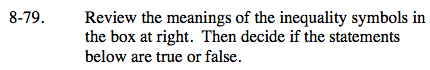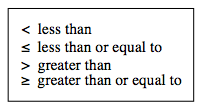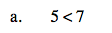Is 5 less than 7?

True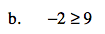Is −2 greater than or equal to 9?

False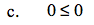Is 0 less than or equal to 0?

True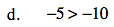Is −5 greater than −10?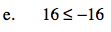Is 16 less than or equal to −16?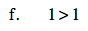Is 1 greater than 1?